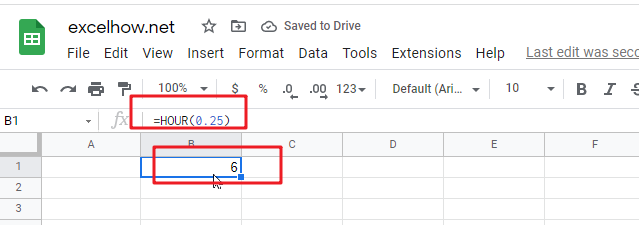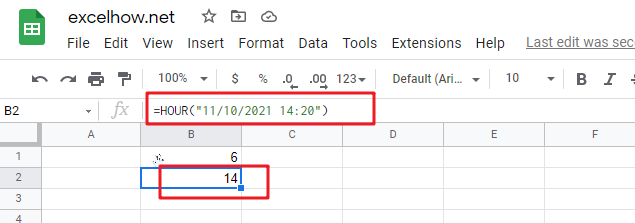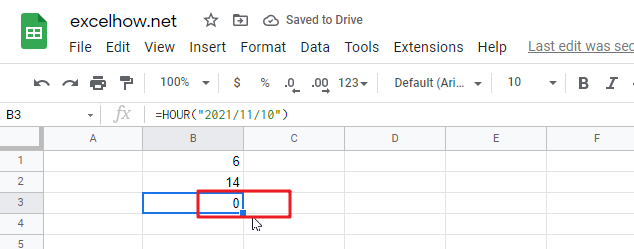This post will guide you how to use Google Sheets HOUR function with syntax and examples.

## Description

The Google Sheets HOUR returns the hour of a time value. Or returns an integer value that represent the hour component of a given time. And the return value is between 0-23.

For example, if you pass a time of 13:36 PM into HOUR function, and it will return 13. So you can use this function to extract the hour component from a time value.

The HOUR function can be used to get the hour component of a time as a number between 0-23 in google sheets. The purpose of this function is to get the hour as a number from a time and its returned values is a number between 0 and 23.

The HOUR function is a build-in function in Google Sheets and it is categorized as a Date function.

## Syntax

The syntax of the HOUR function is as below:

=HOUR (serial_number)

Where the HOUR function arguments is:

• Serial_text -This is a required argument. The time from which you want to extract the hour component. it may be typed as text string within quotation marks (for example, “13:52 PM”), or it can be a decimal numbers(for example, 0.5), or the result of a formula (for example, TIMEVALUE(“13.54PM”))

Note:

• Time values are a portion of a date value and represented by a decimal number (for example, 12:00 PM is represented as 0.5 because it is half of a day).
• A serial date is how the Microsoft Excel stores dates and times and it represents the number of days since 1900-01-01, so the January 1, 1900 date is serial number 1 by default.
• if the serial_number is not a valid Excel time, it will return #VALUE! error.

## Google Sheets HOUR Function Examples

The below examples will show you how to use google sheets HOUR Function to return the hour of a given time value.

1# get the hour component of 25% of 24 hours,, enter the following formula in Cell B1.

`=HOUR(0.25)`2# get the hour portion of the date and time value, enter the following formula in Cell B2.

`=HOUR("11/10/2021 14:20")`3# Get the hour portion of the date with no time portion specified, enter the following formula in Cell B3.

`=HOUR("2021/11/10")`so it will be considered 12:00AM or 0 hours.